# PHY138Y - Mechanics - Class 10 - Monday October 16, 2006

## Introduction

" Science is facts; just as houses are made of stones, so is science made of facts; but a pile of stones is not a house and a collection of facts is not necessarily science."

-- Poincaré

## Today's Class

We finished Chapter 9 by introducing the angular momentum, and then made a good start on Chapter 10 - Energy. I diverged from the textbook a few times in class today, and therefore will review that material a little more carefully than usual below.

### The Rotational Analog of Mass

Although the text doesn't do it until Chapter 13, I thought it would be worthwhile to discuss the fact that the linear momentum is:

p = mv

while the angular momentum can be writte as:

L = mr2Both of these are some quantity involving the mass times a speed. This leads us to suspect, correctly as it turns out, that the quantity mr2 will appear in our equations for rotational motion in the same that that the mass appears in the corresponding equations for linear motion.

### Tennis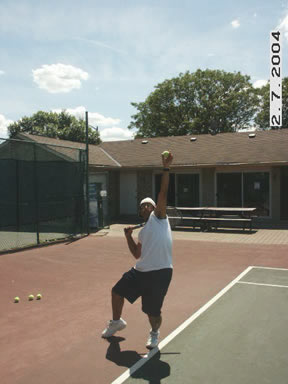The above photographs are of Amin, a PHY138 student from three years ago, serving a tennis ball.

In order to maximise the speed of the serve, Amin needs to get the part of the racket that contacts the ball going at the highest possible speed. He does this with four lever arms:

1. He cocks his wrist before the serve, and then rotates his hand and the racket about his wrist.
2. He rotates his forearm and wrist about his elbow.
3. He rotates his upper arm and elbow about his shoulder.
4. He rotates his entire body including his shoulder about the tip of his left foot.

Note that at contact, all four of these levers are at the maximum values as he "uncoils" into the serve. For each, the speed about the axis of rotation is given by:r

where the angular speeds and radii are different numbers for each of the four rotations. Of course, except for the fourth rotation, the pivot point is also moving at the moment of impact.

Thus the speed of the racket as it contacts the ball is as large as possible. One of the suggested problems from Chapter 9 explores the tennis serve further.

### In-Class Question

I asked the text's Stop To Think 9.6. About 75% of the class got this question correct. Reminder: it is really worth your while to do all of the Stop To Think questions in the text and be sure you understand why the correct answer is correct.

### §10.1

In this section the text has a lovely introduction to the concept of energy as a "natural money." We didn't discuss this in class.

The origins of this treatment go back to at least Richard Feynman, who was a master teacher and also won a Nobel Prize in physics.

 I recently wrote a little document with much in common with both Knight and Feynman's approach. If you are interested, it is available via the link to the right.### Demonstration & Flash Animations

 We did a demonstration in class. A Flash animation of the the demonstration is available. We asked the class to predict that results of the demonstration before we did it.80% of the class answered C: they arrive at the same time. About 20% of the class answered A: the ball on the straight track arrives first.

The demonstration, as well as the Flash animation, show that in fact the right answer is B: the ball on the curved track "wins" the race. Only 13 students gave this answer.

 Some people find that thinking about the problem in terms of skiers instead of balls on a track is helpful. A Flash animation of this case is also available.### The Gravitational Field

In class I introduced the concept of a field. Since this is not in the textbook, here I will review that discussion.

 In Newton's original description of gravitation, the Earth exerts a force w on a mass m as a long-range action-at-a-distance interaction. However, it is possible to break the description of this interaction into 2 parts: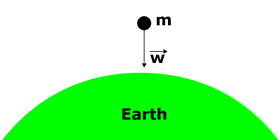1. The Earth creates a gravitational field Eg at all regions of space around it.
2. If a mass m is placed at some point in space, the gravitational field at that point exerts a force on the mass.

It turns out that the simplest possible definition of Eg is best. If, as usual, we choose the y axis to point upwards, then near the surface of the Earth we say the gravitational field is:Then the force exerted on a mass is just the mass times the field: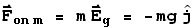This approach to forces will be used heavily in the 3rd Quarter of PHY138.

But we can begin to see the value of a field description now. We have been talking about the gravitational potential energy, and have also been discussing how the overall energy of an isolated system is conserved. But exactly what is the gravitational potential energy? It turns out that it is just as real as the other forms of energy, and is stored in the gravitational field.

### Almost §11.2

At the end I did a derivation very similar to one in Chapter 11, whose result I will use at the beginning of our next class. Here is an overview of that derivation. If you are taking MAT135 you will discover that in addition to an important Physics result, I am also introducing some facts about integration.

 We will be building on a Flash animation we used way back in Class 4. For your convenience, here is another link to that animation.First we consider a plot of force versus distance as shown. We construct three rectangles of equal widths, each with a height equal to the average value of the force for that rectangle. You can probably already anticipate that I am going to end up increasing the number of rectangles and taking the limit ass goes to zero to get the area under the curve.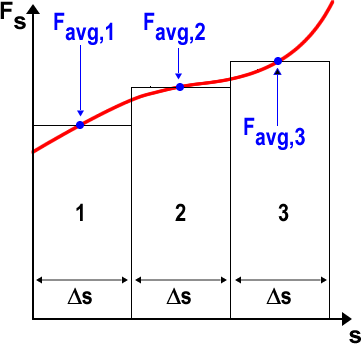Ifs is reasonably small, then the average acceleration of the object in region 1 is as given by the kinematics for constant acceleration: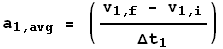(1)

We can similarly write a simple expression for the average speed in region 1:(2)

From this we can write that the distance travelled is just the average speed tiimes the time: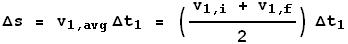(3)

Now we use Newton's 2nd Law and Eqn. 1 to get:(4)

We use this and Eqn 3 to find the area of rectangle 1.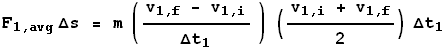(5)

The times cancel out, so with some trivial algebra we get:(6)

We can go through the same steps for region 2 to get: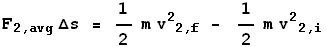(7)

When we add up the areas of regions 1 and 2, we get a cancellation because v2,i is equal v1,f so: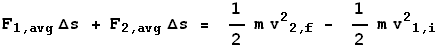(8)

Adding the third region to the sum, increasing the number of rectangles to infinity in the limit ass goes to zero we end up having proven that:

 The total area under a force versus distance plot is just the change in kinetic energy.

### Other Class Materials

 Pdf version of the PowerPoint on the side screens.Today's Journal.## Suggested Problems

• Chapter 10: 10, 15, 42, 54The arrows let you jump to the previous/next class summaries.